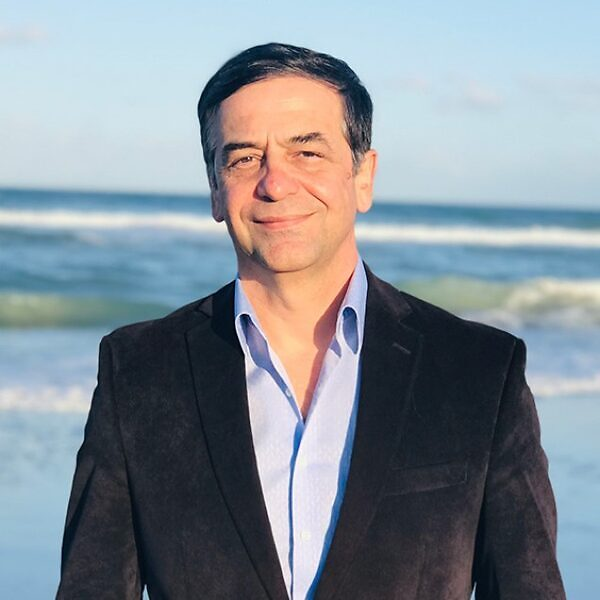# Pontryagin Maximum Principle in optimal control of coherent spin dynamics of radical pairs in Quantum Biology I. Mathematical foundation and optimality conditions.### Date

Wednesday, July 26, 2023 - 14:00 to 14:45

B250

### Description

Lecture 4. Prof. Ugur Abdulla

Title: Pontryagin Maximum Principle in optimal control of coherent spin dynamics of radical pairs in Quantum Biology I. Mathematical foundation and optimality conditions.

Abstract: The aim of this project is to develop the mathematical foundation  of quantum optimal control with the ultimate goal of understanding fundamental mechanisms

connecting atomic and cellular levels in quantum biology. Optimal control of the external electromagnetic field and internal hyperfine parameters for the maximization of the quantum singlet-triplet yield of the radical pairs in biochemical reactions modeled by Schrödinger system with spin Hamiltonians given by the sum of Zeeman interaction and hyperfine coupling interaction terms are analyzed. First- and second-order Fréchet differentiability in Hilbert spaces is proved and the formula for the first- and second-order Fréchet derivatives are derived. Pontryagin Maximum Principle (PMP) is proved and the band-bang structure of the optimal control is established. A closed optimality system for the identification of the bang-bang optimal control is revealed. It consists of system of nonlinear ODEs for finding the optimal state vector and its adjoined, and with subsequent identification of the bang-bang optimal control via PMP. Several numerical algorithms are developed for solving nonlinear optimality system. PMP and bang-bang optimal control reveal the natural ability of the system to exist in several distinct states simultaneously, such as inter system crossing between singlet-triplet states. The results form a mathematical framework for computational and experimental applications.

All-OIST Category: